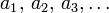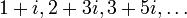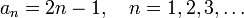# Sequence

A sequence, in mathematics, is an enumerated list; the elements of this list are usually referred as to the terms. Sequences may be finite or infinite.

Formally, given any set X, an infinite sequence of its elements is a function (f, say) defined on the natural numbers {1,2,3,...}, with values in X. Similarly, a finite sequence is a function f defined on {1,2,3,...,n} with values in X. (We say that n is the length of the sequence).

In a natural way, the sequences are often represented as lists:$a_1,\, a_2,\, a_3,\dots$

where, formally, a1 = f(1), a2 = f(2) etc. Such a list is then denoted as (an) or (an)n, with the parentheses indicating the difference between the actual sequence and a single term an.

Some simple examples of sequences of the natural, real, or complex numbers include (respectively)

10, 13, 10, 17,....
1.02, 1.04, 1.06,...$1 + i, 2 + 3i, 3 + 5i,\dots$

Often, sequences are defined by a general formula for an. For example, the sequence of odd naturals can be given as$a_n = 2n - 1,\quad n=1,2,3,\dots$

There is an important difference between the finite sequences and the sets. For sequences, by definition, the order is significant. For example the following two sequences

1, 2, 3, 4, 5 and 5, 4, 1, 2, 3

are different, while the sets of their terms are identical:

{1, 2, 3, 4, 5} = {5, 4, 1, 2, 3}.

Moreover, due to indexing by natural numbers, a sequence can list the same term more than once. For example, the sequences

1, 2, 3, 3, 4, 4 and 1, 2, 3, 4

are different, while for the sets we have

{1, 2, 3, 3, 4, 4} = {1, 2, 3, 4}.

## Basic definitions related to sequencesSome content on this page may previously have appeared on Citizendium.